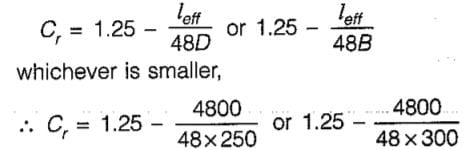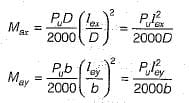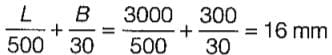Test: Columns - 1

# Test: Columns - 1

Test Description

## 10 Questions MCQ Test GATE Civil Engineering (CE) 2023 Mock Test Series | Test: Columns - 1

Test: Columns - 1 for Civil Engineering (CE) 2022 is part of GATE Civil Engineering (CE) 2023 Mock Test Series preparation. The Test: Columns - 1 questions and answers have been prepared according to the Civil Engineering (CE) exam syllabus.The Test: Columns - 1 MCQs are made for Civil Engineering (CE) 2022 Exam. Find important definitions, questions, notes, meanings, examples, exercises, MCQs and online tests for Test: Columns - 1 below.
Solutions of Test: Columns - 1 questions in English are available as part of our GATE Civil Engineering (CE) 2023 Mock Test Series for Civil Engineering (CE) & Test: Columns - 1 solutions in Hindi for GATE Civil Engineering (CE) 2023 Mock Test Series course. Download more important topics, notes, lectures and mock test series for Civil Engineering (CE) Exam by signing up for free. Attempt Test: Columns - 1 | 10 questions in 30 minutes | Mock test for Civil Engineering (CE) preparation | Free important questions MCQ to study GATE Civil Engineering (CE) 2023 Mock Test Series for Civil Engineering (CE) Exam | Download free PDF with solutions
 1 Crore+ students have signed up on EduRev. Have you?
Test: Columns - 1 - Question 1

### The effective length of a column in a reinforced concrete building frame, as per IS:456-2000, is independent of the

Detailed Solution for Test: Columns - 1 - Question 1

Effective length of a column depends upon:
(i) Flexural stiffness (El/L) of beams joining at a point.
(ii) Flexural stiffness (El/L) of columns joining at a point.
(iii) Type of frame (sway or non-sway).

Test: Columns - 1 - Question 2

### Lateral ties in RC columns are provided to resist

Test: Columns - 1 - Question 3

### The reduction coefficient of a reinforced concrete column with an effective length of 4.8 m and size 250 mm x 300 mm is

Detailed Solution for Test: Columns - 1 - Question 3

In the working stress method the design of a long column is made by considering reduced stresses obtained by multiplying the permissible stresses by a coefficient,= 0.85 or 0.92
So the reduction coefficient is 0.85

Test: Columns - 1 - Question 4

Which of the following statements is incorrect?

Test: Columns - 1 - Question 5

The ratio of the maximum and minimum percentage of longitudinal reinforcement that can be provided in a column is

Detailed Solution for Test: Columns - 1 - Question 5

Maximum reinforcement = 6%
Minimum reinforcement = 0.8%

Ratio = 6/0.8 = 7.5

Test: Columns - 1 - Question 6

In a Pedestal, the factor by which the effective length should not exceed the least lateral dimension is

Detailed Solution for Test: Columns - 1 - Question 6

Very short columns with effective length less than three times least lateral dimension are called pedestal columns.

Test: Columns - 1 - Question 7

A reinforced concrete beam of 10 m Effective span and 1 m effective depth is supported on 500 mm x 500 mm columns. If the total uniformly distributed load on the beam is 10 MN/m, the design shear force for the beam is

Detailed Solution for Test: Columns - 1 - Question 7

The shear force should be calculated at critical section i.e. 1 m away from the face of the column. Effective span of beam is to be taken as the clear span plus effective depth of beam or centre to centre spacing of supports which ever is less. The location for shear calculation will be,
x = 0.25 + 1 = 1.25 m
∴ Design shear force = (10 x 10)/2 - 10 x 1.25
= 37.5 MN

Test: Columns - 1 - Question 8

Which of the following are the additional moments considered for design of slender compression member in lieu of deflection in x and y directions?

where Pu is axial load; lex and ley are effective lengths in respective directions; D is depth of section perpendicular to major axis; b is width of the member)

Detailed Solution for Test: Columns - 1 - Question 8Test: Columns - 1 - Question 9

What is the minimum number of longitudinal bars provided in a reinforced concrete column of circular cross-section?

Detailed Solution for Test: Columns - 1 - Question 9

As per IS : 456-2000 clause 26.5.3.1 (a) the minimum number of longitudinal bars provided in a column shall be four in rectangular columns and six in circular columns.

Test: Columns - 1 - Question 10

An axially loaded column is of 300 mm x 300 mm size. Effective length of column is 3 m. What is the minimum eccentricity of the axial load for the column?

Detailed Solution for Test: Columns - 1 - Question 10

Minimum eccentricity equals to unsupported length of column/500 plus lateral dimension/30, subject to a minimum of 20 mm.So eccentricity = 20 mm

## GATE Civil Engineering (CE) 2023 Mock Test Series

25 docs|282 tests
 Use Code STAYHOME200 and get INR 200 additional OFF Use Coupon Code
Information about Test: Columns - 1 Page
In this test you can find the Exam questions for Test: Columns - 1 solved & explained in the simplest way possible. Besides giving Questions and answers for Test: Columns - 1, EduRev gives you an ample number of Online tests for practice

## GATE Civil Engineering (CE) 2023 Mock Test Series

25 docs|282 tests